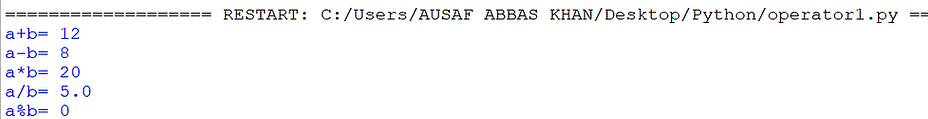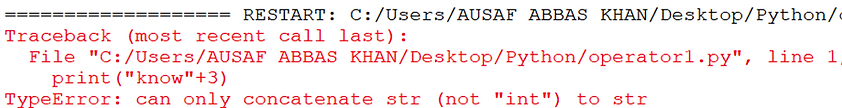top of page## Python: Operators

Arithmetic Operators

arithmetic operator

Example:

a=10

b=2

print (‘a+b=‘,a+b)#12

print (‘a-b=‘,a-b)#2

print(‘a*b=‘,a*b)#20

print(‘a/b=‘,a/b)#5.0 ---- represents answer in float

print(‘a%b=‘,a%b)#0

print(‘a**b=‘,a**b)#10^2=100Note:

• Division operator always generates floating point value irrespective of whether arguments are int  or float.• Floor division represents answer in int (if both argument are int) and float (if both argument are float).• + (addition) operator is applicable for str type also which is called string concatenation. For string concatenation both argument must be string only.

For example: print("know"+"cubs")If we try to execute: print("know"+3)String Multiplication  operator:

* (multiplication) operator acts as string multiplication operator or string repetition operator. For this, one argument should be string and other should be int.

For exampleRelational Operators

Relational operator

Note: We can apply relational operator with number, string and boolean types.

Example 1:

a=20 , b=10

print (‘a>b:‘,a>b)#True

print(‘a<b:‘,a<b)#False

print(‘a>=:b=‘,a>=b)#True

print(‘a<=:b=‘,a<=b)False

Example 2:

a=’salman’  ,b=’amir’

print (‘a>b:‘,a>b)#True

print(‘a<b:‘,a<b)#False

print(‘a>=b:‘,a>=b)#True

print(‘a<=b:‘,a<=b)False

We can apply relational operator for string also. Comparison  is based on alphabetical order or Unicode value.

Example 3:

a=True   b=False

print (‘a>b:‘,a>b)#True

print(‘a<b:‘,a<b)#False

print(‘a>=b:‘,a>=b)#True

print(‘a<=b:‘,a<=b)#False

We can apply relational operator for bool type also. Internally True treated as 1 and False treated as 0.

Chaining of Relational operator:

If every comparison is True result is True.

For example

10<20<30<40<50  #True

10<20 True

20<30 True

30<40 True

40<50  True

Hence result is True.

If at least one condition is False then result is False.

For example

10<20<30<40<50>60 #False

10<20 True

20<30 True

30<40 True

40<50  True

50>60 False

Hence result is False.

Equality operators (== and  !=):

• It compares only content not address.

• If content is same then True, otherwise False.

Example:

10==20 #False

10!=20 #True

10==True #False

‘mujtaba’==’mujtaba’ #True

10==’mujtaba’ #False

Chaining of ==

If every comparison is True result is True.

For Example

10==20==30==40==50 #False

10==20#False

20==30#False

30==40#False

40==50#False

Hence result is False.

10==5+5==3+7==2*5 #True

10==5+5#True

5+5==3+7#True

3+7===2*5#True

Hence result is True.

Some Important Examples:

1. ‘a’==97 #False (incompatible Types)

2. (10+2j)==(10+2j) #True

3. (10+2j)==’durga’ #False (incompatible Types)

4. 10==10.0 #True

5. 10==10.1 #False (int value is changed to float then comparison takes place)

6. 10==’10’ #False(incompatible Types)

7. 1==True #True (Internally True is treated as 1)

8. 10.10==10.1 #True

Note: Equality operator never gives an error, it checks content only. On comparison of incompatible types, result will be 'False'.

Logical Operators (and, or, not)

• and ==> result will be True if both arguments  are True.

• or ==> result will be True if at least one argument is True.

• not ==> result will be True if argument is False and False if argument is True.

For boolean types: result is always boolean.

Example:

True and True # True

True and False #False

True or False #True

True or True #True

not True #False

not False #True

Logical operatorsFor non-boolean types: There are some rules for non-boolean types.

• 0 means False.

• non-zero means True.

• empty string means False.

x and y

If x evaluates to False then result is x otherwise  y.

Example:

0 and 20 #0

Here x=0 means False, hence result is x i.e 0.

Example:

10 and 20 #20

Here x=10 means True hence result is y i.e 20.x or y

If x evaluates to True then result is x otherwise y.

10 or 20 ==>10

Here x=10 means True hence result is x i.e 10.

0 or 20 ==>20

Here x=0 means False hence result is y i.e 20.

0 or 0==>0

Here x=0 means False hence result is y i.e 0.

0 or True ==>True

Here x=0 means False hence result is x i.e 0.

10 or 10/0 ==> 10

0 or 10/0 ==>divide by 0 errornot x

If x evaluates to True then result is False and if x evaluates to False then result is True.

not 10 ==>False

not ‘’” ==>True

not 0 ==> TrueBitwise Operators (&,|,^,~,<<,>>)

• Applicable only for int and  bool type, if we try for other types we will get error.

• Comparison takes place bit by bit.

4&5 #valid

True &True #valid

10.5 & 2.6 #invalid (unsupported operand type for &)

Bitwise operator& : if both bits are 1 then only 1 otherwise 0

Example:

4&5 ==>100&101 ==>100 ==>4| : if at least one bit is 1 then 1 otherwise 0

Example:

4|5 ==>100|101 ==>101==> 5^ (xor ) : if both bits are different then 1 otherwise 0

Example:

4^5 ==>100^101 ==>001 ==>1~ : bitwise complement operator

Example:

~4If you think answer is 3 then you are  wrong.Correct answer is -5 but how we get it?Example:

~True#-2<< : bitwise left shift (right hand side vacant cells fill with 0s)

Ex :  10<<2==> 40>> : bitwise right shift (left hand side vacant cells fill with sign bit)

Example:

10>>2Assignment Operators (=)

Assignment operators are used to assign values.

For example: x=10

A int value of 10 is assigned to x.

Note: Increment (++) and decrement (–-) operator are not available in python, if you use it will give error.

Assignment operatorCompound assignment

+=,-=,*=,/=,%=,**=,//=

Ternary Operator: (?:)

It is also known as conditional operator.

Syntax of ternary operator in other languages:

x=(condition)?firtsValue:secondvalue

x=first value if condition, else second Value

Syntax of ternary operator in Python:

x=first value if condition else second value.

Example:

a,b=10,20

x=30 if a>b else 40

print(x) ==>40

Read two numbers from keyboard and print min valueRead three numbers from keyboard and print max valueSpecial Operators

1. Identity operators (is ,is not)

2. Membership operators (in ,not in)

Identity operators

Is and is not compares the address of objects. If the objects are same it returns True, otherwise False.

Example 1:

A=10

B=10

Print (a is b) ==>True

Example 2:

List1= [10, 20, 30]

List2= [10, 20, 30]

Print (List1 is List2) ==>False

Print (List1 == List 2) ==>True

Content of  List1 and List2 is same but they are pointing to different objects. Hence is returns False (reference comparison)  and == returns True (content comparison).

Specil operatorMembership operators:

This operator is used to check membership of an element.

Example 1:

List1= [10, 20, 30]

Print (10 in list1) #True

Print (70 not in list1) #True

Example 2:

s=”Hello Learning python is very very easy!!!”

Print (‘Hello’ in s) #True

Print(‘L’ in s) #True

Operator Precedence in Python

Operator precedence means in which order operators will be evaluated. Following is a general order for operator evaluation:

• () highest priority goes to parenthesis in python.

• ** exponential operator  or power operator

• ~ unary operator

• /,%,//

• +,-

• <<,>>

• &

• ^

• |

• >,>=,<.<=,==,!=

• =,+=,-+,*=

• is , is not

• in , not in

• not

• and

• or

Example:

a=30

B=20

C=10

D=5

Print((a+b)*c/d) #100.0

Print((a+b)*(c/d)) #100.0

Print(a+(b*c)/d) #70.0

Operator precedence
bottom of page